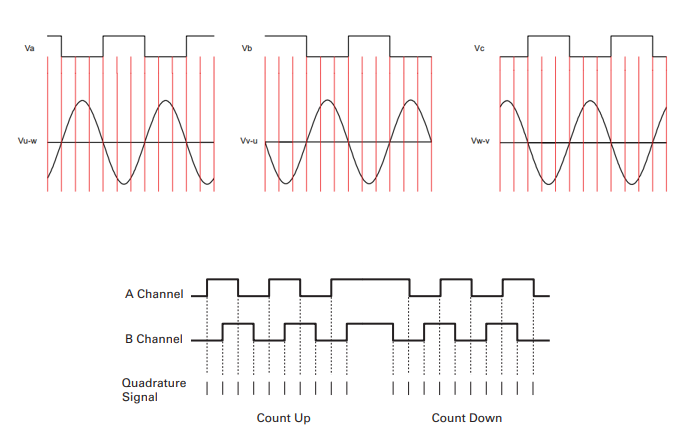Quadrature A/B Encoder can be used in combination with Hall sensors to achieve the optimal motor's commutation. Hall sensors can give the absolute position of the rotor* but have small resolution and quadrature encoders have higher resolution but do not provide any reference point**. The combination of both sensors ensures that the motor will be commuted with the highest resolution and without the need for a zero point search every time the controller is powered on, as it happens when quadrature encoder is used alone.

Below are the basic steps required to run a motor in Open Loop by using its Hall sensors. Only the necessary information to run the motor will be provided, so for a more detailed explanations please refer to the Roboteq Controllers User Manual.

* Applicable only if the motor has 1 pole pairs. In other case the position is known only in reference to the angle of the electrical field that will be provided.

** Z signal is not used in Roboteq controllers but even if it was used it cannot become useful for the commutation of the motor.

Physical connections

The Hall sensors are always connected to the Molex connector of the controllerThe encoder sensors are connected to different pins of the DB25 connector, depending on the controller model. Consult the controller's datasheet or the controller's pinout in Roborun+ Utility to find the exact pinssignal of channel 1 connects to ENC1A pin
signal of channel 1 connects to ENC1B pin

signal of channel 2 connects to ENC2A pin

signal of channel 2 connects to ENC2B pin

To run the motor in Open Loop with Hall and Encoder sensors follow the next steps:

1. Configure the Molex Input to “Hall Sensors”

SSI sensors and Hall sensors share the same Molex conenctor, so the controller must know the type of sensor is expecting to find there.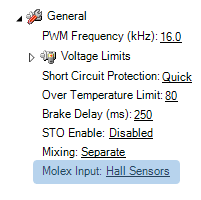2. Configure the number of “Pole Pairs”

Pole pairs parameter is essential for the sinusoidal commutation and for the speed calculation.

Pole pairs is the mumber of motor poles divided by 2.3. Configure the switching mode to sinusoidal

Roboteq controllers support two ways of supplying current to the motor: Trapezoidal and Sinusoidal. In sinusoidal mode the current is sinusoidal so it has less harmonics and the motor is driven in a smoother and quiter way, developing the minumun torque riple. Since sinusoidal commutation is superior to Trapezoidal, this method should be configured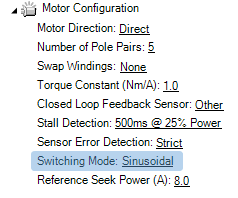4. Set the Reference seek power to the rated current of the motor

.

The reference seek power is the current that will be provided to the motor during the motor/sensor calibration process. If the reference current is too low or too high the motor/sensor setup will not be performed correctly.5. Configure the sinusoidal angle sensor to Hall+Encoder Sensor

This is the sensor that will be used to get the angle of the rotor. Knowing the exact angle of the rotor each time is necessary to make it rotate.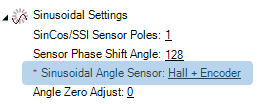6. Configure the Encoder PPR and channel

The PPR (Pulses per revolution) parameter can be obtained from the encoder datasheet. Make sure that the PPR is configured correctly as it is an essential parameter for the commutation of the motor. The channel that the encoder will be used should be configured as well. The action of the encoder (e.g. Feedback) is not necessary to be configured yet, but it can be set as "Feedback" if the encoder will be used for the Closed Loop operation as well.7. Configure the FOC Flux and Torque gains

These gains concern the two PI controllers of the Current Loop, regulating the Torque and Flux current. The proper control of the Torque and Flux current is essential to achieve the optimal commutation and safe operation of the motor. The Current Loop can be tuned by knowing the Resistance and Inductance parameters of the motor. So there are two options:

Use the datasheet Parameters:

The datasheet parameters will give the phase-to-phase or the phase Resistance and Inductance values.

• If the parameters are phase-to-phase values they should be divided by 2
• All values should be changed to original units (Ohm and Henri and not mOhm and uH that are expressed usually)

Then the Flux and Torque gains can be calculated as follows:

Flux P gain = 314 * Phase Inductance (H)

Flux I gain = 314 * Phase Resistance (Ohm)

Torque P gain = 314 * Phase Inductance (H)

Torque I gain = 314 * Phase Resistance (Ohm)

Example:

p-p Resistance: 400 mOhm

p-p Inductance: 200 uH

Flux P gain = 314 * 0.000100 (H) = 0.0314

Flux I gain = 314 * 0.2 (Ohm) = 62.8

Torque P gain = 314 * 0.000100 (H) = 0.0314

Torque I gain =  314 * 0.2 (Ohm) = 62.8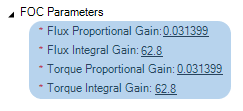Tha value of 314 will give a fixed Bandwidth of 50 Hz. For more information about the FOC tuning refer to the Roboteq Controllers User Manual.

Use the Motor characterization Tool:

The Roboteq controller has a tool that can estimate the motor parameters and calculate the FOC values. This should be used only in case that the motor parameters are not known, becasue the estimated values might differ from the real.

To run the motor characterization go at diagnostics tab and click the motor characterization button. The motor will start making some noise.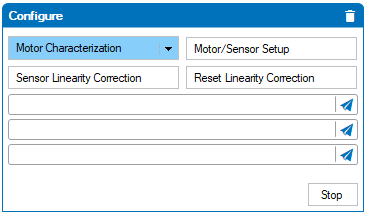When asked select a Banwidth of 50 Hz. the controller will clalculate the FOC gains andautomatically save the FOC parameters.

8. Configure the Amps Limit parameter

The controller will prevent the motor current from exceeding the amp limit parameter by reducing the motor voltage. Please note that although this is a sufficient process, it does not act immediately so it cannot prevent any current spikes. This value should be set equal to the peak current of the motor.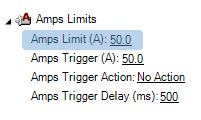Since the peak current of a motor is only allowed for a specific amount of time, Roboteq controller offers the option to trigger an action if the motor current exeeds a value for an amount of time. This can be configured by the Amps Trigger parameter.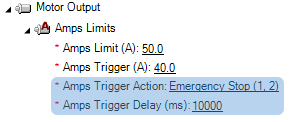9. Configure the Operating Mode to “Open Loop”

Even if the final target is to use Close Loop Speed or Position mode, it is mandatory to first test the motor in Open Loop.10. Go to Diagnostics tab and run the Motor/Sensor Setup

Motor/Sensor setup is the process where the controller will record the locations of the Hall sensors in positive and negative direction. This is required for the Sinusoidal commutation of the motor. During the calibration, the motor will move for some degrees in both directions.Important notice! The motor shaft should be free to rotate and without load

In the chart enable the Motor amps and Hall Status parameters and ensure the following:

• Hall status Min and max is 1 and 6
• Hall status takes all the intermediate values including 1 and 6
• Hall status 7 and 0 values are not present
• The Motor Amps are equal to the Reference seek power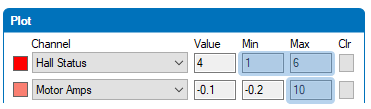When the motor/sensor setup finish it will print the Hall Sensor Angle Table (HSAT). Make sure that the HSAT has not repeated values and there is not any 64 value.Repeat the motor/sensor setup a few times and confirm that the HSAT results are consistend. A deviation up to 5 degrees is acceptable, but a higher deviation might denote that the reference seek power is not set properly.

Important notice! Sensor Error Detection should be disabled in the unused channel

At firmware version 2.1 or later, the sensor error detection method is implemented. This will trigger the motor sensor error if one of the HALL sensors is disconnected. I dual channel controllers, if one of the two channels is not used, please disable the sensor error detection.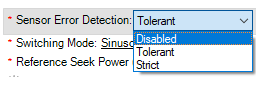11. Evaluate Open Loop Operation

Now the motor should be able to rotate in Open Loop with Sinusoidal commutation. Go at the run tab and move the slidebar slowly until the motor start rotating. If the motor is able to rotate, evaluate the following:

• Run the motor in both directions. Verify that the motor amps value is below the “no load current” of the motor, when the given command is constant. The motor current can increase during acceleration/deceleration but at constant power, the motor amps should be low.
• Give full command in both directions. Verify that the Motor amps in both CW and CCW direction is symmetrical. If the current is very different (more than 1 A) then something in the configuration might not be correct.
• Verify that the Motor Flux amps parameter is has a small fluctuation and is stabilizes quickly to zero when the motor accelerates/decelerates.
• Verify that the FOC angle correction parameter is stable or that it stabilizes quickly by changing the motor speed
• Verify that the Internal Sensor Speed RPM is stable when giving a constant command
• Verify that the Internal Sensor Speed RPM is symmetrical when giving full command in both directions. Depending on the motor, an error up to 5% can be acceptable, but higher variation might denote a tunning or motor issue.
• Verify that the Intarnal Sensor Speed RPM and Encoder Speed RPM have similar values at all cases. If the Encoder and Internal RPM are different maybe some parameters are not configured properly
• Verify that the Internal Sensor Speed RPM  is positive by giving a positive motor command. If not, invert the pole pairs sign, to fix that.
• Verify that the Encoder Speed RPM is positive by giving a positive motor command. If not, invert the RPM parameter sign to fix that.Important notice! Motor speed should be positive by giving a positive command

If the motor speed is inverted, the motor will run uncontrolled when configuring the Closed Loop.

If the Internal Speed RPM is opposite, inver the sign of motor pole pairs (e.g. from 5 make it -5) to fix itIf the Encoder Speed RPM is opposite, invert the Encoder PPR sign to fix it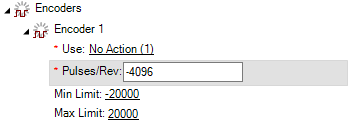If all the tests have passed succesfully then the motor is ready to be configured in Closed Loop!

If not, please Open a technical support ticket at our Helpdesk platform.